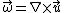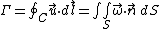# vorticity

Also found in: Dictionary, Thesaurus, Medical, Wikipedia.

## Vorticity

A vector proportional to the local angular velocity of a fluid flow. The vorticity, , is a derived quantity in fluid mechanics, defined, for a flow field with velocity , by Eq. (1).

(1)As the curl of the velocity vector, the vorticity is a vector with the dimensions of both a frequency and an angular velocity . The component of vorticity along a particular axis is related to the rate of rotation of the fluid about the axis. For this reason, flows for which are described as irrotational. See Dimensional analysis, Velocity

#### Circulation

Closely related to vorticity is the fluid circulation, &Ggr;, defined, for any closed contour, C, in a fluid, by Eq. (2).

(2)In this definition, indicates the conventional counterclockwise contour integral around the contour C, is a unit vector tangent to the contour, and S is an arbitrary curved surface bounded by the contour C. [The equality of the two integrals in Eq. (2) may be deduced by the application of Stokes' theorem.] The circulation is thus a scalar quantity equal to the integrated component of vorticity normal to the surface around which &Ggr; is taken. Circulation is important because the Kutta-Joukowski law of aerodynamics states that the lift generated by a two-dimensional airfoil is . In this expression, is the fluid density, U is the free-stream velocity, and &Ggr; is the bound circulation of the airfoil, defined conventionally as the negative of the definition above. See Aerodynamics, Airfoil

#### Vortex line and vortex tube

A vortex line is defined as a line that is everywhere tangent to the local vorticity vector (analogous to a streamline). A series of adjacent vortex lines is referred to as a vortex tube. The first Helmholtz vortex law states that at any instant in time the circulation about all loops taken around the exterior of a vortex tube is the same. Thus, vortex tubes must either form loops entirely within a fluid or terminate at some fluid boundary. See Vortex

#### Kelvin's theorem

Kelvin's theorem considers how the circulation &Ggr; around a material loop in a fluid (a loop that moves with the fluid) varies in time. Starting with the Navier-Stokes equations, Lord Kelvin showed that if (1) the fluid is inviscid along the loop, (2) the fluid is subject only to potential body forces, and (3) the fluid pressure is a function of density alone, then the rate of change of &Ggr; is 0. In other words, the circulation around a material loop is time-independent. Kelvin's theorem may also be stated slightly differently: subject to the above three constraints, vortex lines are material lines, convected with the local fluid velocity. See Kelvin's circulation theorem

#### Generation

Kelvin's theorem can tell what happens when vorticity is already present in a flow, but it sheds no light on how vorticity is generated. To answer this question, it is useful to consider situations for which Kelvin's theorem is inapplicable: flow with viscosity, with nonpotential body forces, and for which the pressure is not solely a function of the density.

The action of viscosity has two effects on vorticity. One effect of viscosity is to cause the diffusion of vorticity in a fluid. A second effect of viscosity is the generation of vorticity at a wall where there is a pressure gradient at the wall. See Viscosity

A common example of a nonpotential body force is the Coriolis force, which is present in a rotating frame of reference. This force generates vorticity in a fluid, and is a major cause of the large-scale circulation in the atmosphere and oceans.

There are many flows for which the pressure may not be solely a function of the density (so-called baroclinic flows), such as the flow of gas with heat addition and the flow of water with salinity variations. Pressure gradients in such flows generate vorticity. This source of vorticity is called baroclinic torque, and is important in atmospheric flow, buoyancy-driven flow, and oceanographic flow.

McGraw-Hill Concise Encyclopedia of Physics. © 2002 by The McGraw-Hill Companies, Inc.

## vorticity

[vȯr′tis·əd·ē]
(fluid mechanics)
For a fluid flow, a vector equal to the curl of the velocity of flow.
McGraw-Hill Dictionary of Scientific & Technical Terms, 6E, Copyright © 2003 by The McGraw-Hill Companies, Inc.

## vorticity

Vorticity is a measure of rotation in the atmosphere, or “spin,” in the air. If the spin, as viewed from above, is counterclock-wise in the Northern Hemisphere, it is called a vorticity cyclonic and given a positive value. If the spin is clock-wise, it is called a vorticity anticyclonic and given a negative value. The opposite conventions apply in the Southern Hemisphere. In forecasting large-scale weather events, one is concerned primarily with “relative vorticity” and focuses on the rotation of air around a vertical axis, much like the rotation of a hurricane over the earth's surface. The vorticity caused by the Coriolis effect is another matter. Consider a large volume of air rotating around a common center just as a very large wheel would spin around its axis. One component of the relative vorticity is due to its pure rotation. The greater the wind speed, or V, in this circular motion and the smaller the radius of rotation, R, the greater the vorticity. The contribution toward relative vorticity from rotation alone is V/R. Because tornadoes have high wind speeds and nominal radii of only hundreds of meters, the vorticity associated with them is very high. Hurricanes have high winds, too, but the radius of rotation is greater, so their relative vorticity is less than for tornadoes. A second source of relative vorticity arises from wind shear, which can be defined as a change in wind speed between two locations. Wind shear in the horizontal imparts a spin to volumes of air caught in the flow. This shear gives rise to cyclonic vorticity because it imparts a counterclock-wise spin to volumes of air caught in the flow. There is a good correlation between large values of vorticity and centers of low pressure in the atmosphere. The association of low pressure and high vorticity with foul weather is a generalization that is quite often true.
References in periodicals archive ?
The distribution of axial, radial and azimythal components of velocity, vorticity, density, and temperature has been calculated using the implicit finite difference method and ADI method.
where [[??].sub.y] denotes the ensemble-averaged y-component of the vorticity vector and [[omega].sub.e] denotes the angular velocity of the crank shaft.
Taking the vorticity magnitude into account, although the peak value on both fine and coarse grids is underestimated, all the calculated data are fairly consistent with the measurements, which is especially obvious on the fine grid.
The advantage of the quaternionic formulation is that, it is better to explain two four-velocities, hydroelectric (Lamb vector), and hydromagnetic (vorticity) fields and the dual Lorenz gauge conditions for dyonic cold plasma.
The cylindrical particles will change the orientation angle under the two torques with which the particles would rotate around its axis of revolution aligned to the vorticity direction when the shear rate is low, while aligning on the flow-gradient plane beyond a critical shear rate value.
Figure 13(c) presents the vorticity plot at the same time instant.
Caption: Figure 14: Isosurface of vorticity (Re = 77 000) for 100 [s.sup.-1] (the color of isosurface represents wind velocity) for AoA=0[degrees]; tilt of the rear rotor nacelles [[delta].sub.t] = 45[degrees].
After finishing, oval vorticity equation can be obtained as follows:
In this previous study, effects of circulation, thickness, and compressibility were retained, while the effects of shed vorticity and downwash were not modeled.
The temporal and spatial characteristics of rear velocity field and vorticity field are contrasted to the PIV testing data as well.
In the section at x/h=0.70, the streamwise vorticity at the top of the nozzle with vortex generator is more evident than that without vortex generator.
The unsteady terrain is more suitable to describe the motion of the fluid state of the earth because of the change of global climate and environment, so the modified models are more rational potential vorticity equations.

Site: Follow: Share:
Open / Close